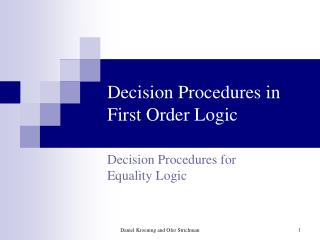DownloadDownload PresentationDecision Procedures in First Order Logic

Decision Procedures in First Order Logic

Télécharger la présentationDecision Procedures in First Order Logic

- - - - - - - - - - - - - - - - - - - - - - - - - - - E N D - - - - - - - - - - - - - - - - - - - - - - - - - - -
Presentation Transcript

1. Decision Procedures in First Order Logic Decision Procedures forEquality Logic Daniel Kroening and Ofer Strichman

2. Outline • Introduction • Definition, complexity • Reducing Uninterpreted Functions to Equality Logic • Using Uninterpreted Functions in proofs • Simplifications • Introduction to the decision procedures • The framework: assumptions and Normal Forms • General terms and notions • Solving a conjunction of equalities • Simplifications      Decision Procedures An algorithmic point of view

3. Basic assumptions and notations • Input formulas are in NNF • Input formulas are checked for satisfiability • Formula with Uninterpreted Functions: UF • Equality formula: E Decision Procedures An algorithmic point of view

4. First: conjunction of equalities • Input: A conjunction of equalities and disequalities • Define an equivalence class for each variable. For each equality x = y unite the equivalence classes of x and y. Repeat until convergence. • For each disequality uv if u is in the same equivalence class as v return 'UNSAT'. • Return 'SAT'. Decision Procedures An algorithmic point of view

5. x4,x5 Example • x1 = x2Æx2 = x3Æx4=x5Æx5x1 x1,x2,x3 Equivalence class Equivalence class Is there a disequality between members of the same class ? Decision Procedures An algorithmic point of view

6. x4,x5 Next: add Uninterpreted Functions • x1 = x2Æx2 = x3Æx4=x5Æx5x1 ÆF(x1)F(x2) F(x1) x1,x2,x3 Equivalence class F(x2) Equivalence class Equivalence class Equivalence class Decision Procedures An algorithmic point of view

7. x4,x5 Next: Compute the Congruence Closure • x1 = x2Æx2 = x3Æx4=x5Æx5x1 ÆF(x1)F(x2) x1,x2,x3 F(x1),F(x2) Equivalence class Equivalence class Now - is there a disequality between members of the same class ? This is called theCongruence Closure Decision Procedures An algorithmic point of view

8. And now: consider a Boolean structure • x1 = x2Ç (x2 = x3Æx4=x5Æx5x1 ÆF(x1) F(x2)) x1,x2 F(x1) F(x2) x4,x5 x2,x3 Equivalence class Equivalence classes case 1 case 2 Syntactic case splitting: this is what we want to avoid! Decision Procedures An algorithmic point of view

9. Deciding Equality Logic with UFs • Input: Equality Logic formula UF • Convert UF to DNF • For each clause: • Define an equivalence class for each variable and each function instance. • For each equality x = y unite the equivalence classes of x and y. For each function symbol F, unite the classes of F(x) and F(y). Repeat until convergence. • If all disequalities are between terms from different equivalence classes, return 'SAT'. • Return 'UNSAT'. Decision Procedures An algorithmic point of view

10. y x z Basic notions E: x = yÆy = zÆzx • The Equality predicates: {x = y, y = z, zx}which we can break to two sets: E= ={x = y, y = z}, E = {zx} • The Equality GraphGE(E) = hV,E=,Ei(a.k.a “E-graph”) Decision Procedures An algorithmic point of view

11. y x z Basic notions 1E: x = yÆy = zÆzxunsatisfiable 2E: x = yÆy = zÇzx satisfiable The graph GE(E) represents an abstraction ofE It ignores the Boolean structure ofE Decision Procedures An algorithmic point of view

12. y x z Basic notions • Dfn: a path made ofE= edges is anEquality Path.we writex =*z. • Dfn: a path made of E= edges +exactly one edge fromE is a Disequality Path. We writex*y. Decision Procedures An algorithmic point of view

13. y x z Basic notions • Dfn. A cycle with one disequality edge is a Contradictory Cycle. • In a Contradictory Cycle, for every two nodesx,yit holds thatx =* yandx* y. Decision Procedures An algorithmic point of view

14. y x z Basic notions • Dfn: A subgraph is called satisfiable iff the conjunction of the predicates represented by its edges is satisfiable. • Thm: A subgraph is unsatisfiable iff it contains a Contradictory cycle Decision Procedures An algorithmic point of view

15. Basic notions • Thm: Every Contradictory Cycle is either simple or contains a simple contradictory cycle Decision Procedures An algorithmic point of view

16. Simplifications, again • Let S be the set of edges that are not part of any Contradictory Cycle • Thm: replacing all solid edges in S with False, and all dashed edges in S with True, preserves satisfiability Decision Procedures An algorithmic point of view

17. Simplification: example x3 • (x1 = x2Çx1=x4) Æ(x1x3Çx2 = x3) • (x1 = x2Ç True) Æ(x1x3Ç x2 = x3) • (:False Ç True) = True • Satisfiable! True False x4 x2 True x1 Decision Procedures An algorithmic point of view

18. Syntactic vs. Semantic splits • So far we saw how to handle disjunctions through syntactic case-splitting. • There are much better ways to do it than simply transforming it to DNF: • Semantic Tableaux, • SAT-based splitting, • others… • We will investigate some of these methods later in the course. Decision Procedures An algorithmic point of view

19. Syntactic vs. Semantic splits • Now we start looking at methods that split the search space instead. This is called semantic splitting. • SAT is a very good engine for performing semantic splitting, due to its ability to guide the search, prune the search-space etc. Decision Procedures An algorithmic point of view